# Characteristic polynomial

of a matrix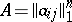over a fieldThe polynomial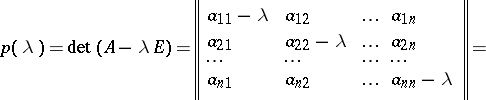over. The degree of the characteristic polynomial is equal to the order of the square matrix, the coefficient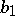is the trace of(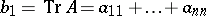, cf. Trace of a square matrix), the coefficientis the sum of all principal minors of order, in particular,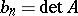(cf. Minor). The equationis called the characteristic equation ofor the secular equation.

The roots of the characteristic polynomial lying inare called the characteristic values or eigen values of. Ifis a number field, then the term "characteristic number of a matrixcharacteristic numbers" is also used. Sometimes the roots of the characteristic polynomial are considered in the algebraic closure of. They are usually called the characteristic roots of. A matrixof orderregarded over an algebraically closed field (for example, over the field of complex numbers) haseigen values, if every root is counted according to its multiplicity. See also Eigen value.

Similar matrices have the same characteristic polynomial. Every polynomial overwith leading coefficient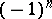is the characteristic polynomial of some matrix overof order, the so-called Frobenius matrix.

For references see Matrix.

The characteristic roots are often also called the eigen values or characteristic values, thereby not distinguishing the roots of the characteristic polynomial inand those in its algebraic closure. Given a polynomial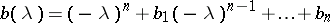. The matrix in companion formwith, has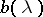as its characteristic polynomial.